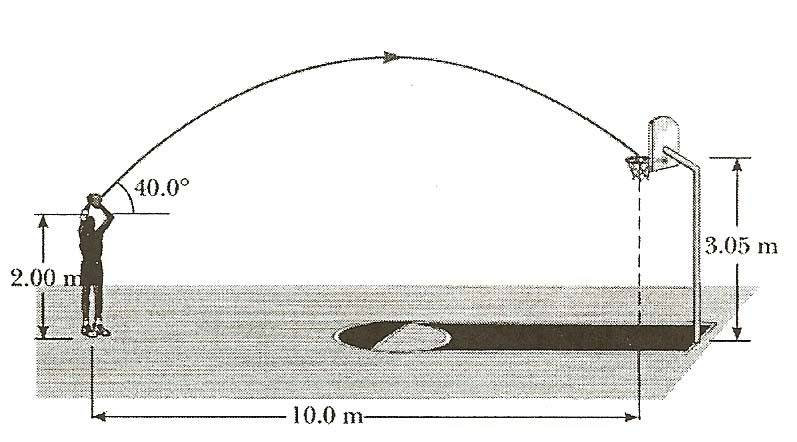## Homework Statement

A basketball player who is 2.00[m] tall is standing on the floor 10.0 [m] from the basket, as in the figure. If he shoots the ball at 40.0degrees angle with the horizontal, at what initial speed must he throw so that it goes through the hoob without striking the backboard? The basket height is 3.05[m].## Homework Equations

xf = xi + vit + 1/2at^2
yf= yi +vit + 1/2at^2

## The Attempt at a Solution

I used the yf equation to come up with an equation that has vi and t as the variables. Then I used the xf equation and solved for t. Then I plugged in that t into the yf equation. It comes out to a pretty large quadratic equation:

-4.9 (10/vi(sin40))^2 + (vi(cos40))(10/vi(sin40)) - 1.05 = 0

Now I'm having trouble finding vi. I'm pretty sure I did everything right, but can someone help me figure out how to solve for vi?

Also, how do you make it so the quations come up looking normal instead of typing it here, it's formatted different? Is that a website you type it in and link here? Thanks!

-Jeff

LowlyPion
Homework Helper

## Homework Statement

A basketball player who is 2.00[m] tall is standing on the floor 10.0 [m] from the basket, as in the figure. If he shoots the ball at 40.0degrees angle with the horizontal, at what initial speed must he throw so that it goes through the hoob without striking the backboard? The basket height is 3.05[m].

## Homework Equations

xf = xi + vit + 1/2at2
yf = yi + vit + 1/2at2

## The Attempt at a Solution

I used the yf equation to come up with an equation that has vi and t as the variables. Then I used the xf equation and solved for t. Then I plugged in that t into the yf equation. It comes out to a pretty large quadratic equation:

-4.9 (10/vi(sin40))^2 + (vi(cos40))(10/vi(sin40)) - 1.05 = 0

Now I'm having trouble finding vi. I'm pretty sure I did everything right, but can someone help me figure out how to solve for vi?

Also, how do you make it so the quations come up looking normal instead of typing it here, it's formatted different? Is that a website you type it in and link here? Thanks!

-Jeff

First of all I hope you didn't show any acceleration in the x direction. I haven't checked what you did, but that term is 0 for Vx.

As to figuring combined x,y speed remember that the x and y components of speed add like vectors.

As to the superscripting and subscripting, I modified your Relevant Equations to show you how to code it. You can also use TEX (LaTex) but that is a more complicated tutorial. If you open up some of the posts with formulas, if you can make a 10 m jumpshot, that won't be so hard.

Last edited: